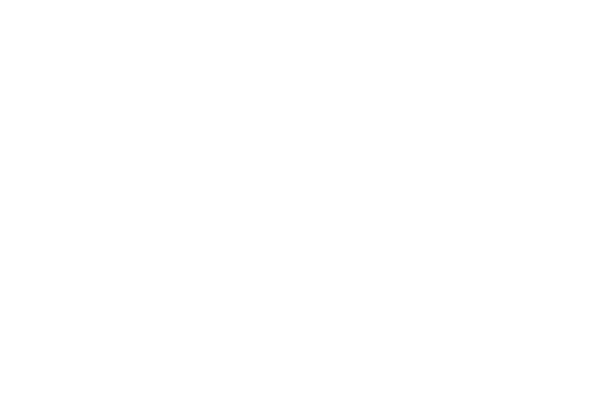### A triangular pyramid with transformations

••••••••••••### Who is online

We have 51 guests and no members online

# A triangular pyramid with transformations

Convertible telescopic geometric teaching and instructional manual on plane geometry and stereometry geometry "Triangular Pyramid"From this model it is possible to obtain all types of a triangular pyramid. Before starting working with models, it is necessary to know that for manipulations and transformations it is necessary to work consistently only with each of the sides separately. The pyramid which shows the theorem of three perpendiculars is of special interest.
SB⊥(ABC)

AC⊥CS follows AC⊥BC and AC⊥BC follows AC⊥CSWith this type of model it is possible to solve numerous tasks. From this model it is possible to receive all types of quadrangles and a triangle for the construction of which it is necessary to make the following: all sides of the basis of a pyramid A, B and C should be pulled, and the edges SA, SB and SC should be decreased in length until they all fit in the plane ofthe triangleABC.

Creation of sectionsThe auxiliary rods are included in the kitfor sections construction, which allow to obtain any sections. It is easy to be convinced, if we notice that the rods can be fixed in any combinations. In a triangular pyramid by means of auxiliary rods, as shown in figure, we demonstrate that section which represents a quadrangle. By the way, it is possible to construct with their help the height, the median, the bisector, etc. Auxiliary rods can also serve for replacement of the broken rods models.

Before passing to the solution of tasks, it should be noted that models are irreplaceable for obtaining drawings of spatial bodieson the background ofthe board. Appropriately holding the modelat the board, it is possible to draw itself as it is visible. For the solution of the tasks concerning a triangular pyramid it is necessary to use a quadrangular pyramid which thanks to the fact that is being modified in various triangular pyramids, gives the chance to demonstrate solutions to specific tasks.We have a triangular pyramid with equal edges which basis is the rectangular triangle.

Height of such pyramid passes through the center of a circumscribed circle.The center of a circle is in the middle of a hypotenuse.We have the pyramid with ribs a, b, c, which are mutually perpendicular. We need to find the volume of a pyramid. The task gets a simple solution if the pyramid is inverted. As we can see, we obtained a pyramid whose base is a right triangle, where the length of basement legs (and the base is a right-angled triangle) and the length of the altitude are known. Thus, the volume of a pyramid is equal and bc/6.To prove that it is possible to inscribe the sphere into any pyramid. This is one of the most difficult problems in stereometry. We wire the cross section which passes through bisector dihedral angle BACD. They intersect in a straight line AH. If we wire the third cross section, then it will intersect with the second cross section in a straight line CM. The point of intersection CH and CM is considered as the center inscribed sphere, as it is equidistant from all sides.